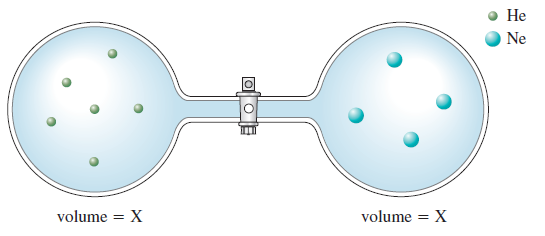# Problem: Consider the flasks in the following diagrams.a. Which is greater, the initial pressure of helium or the initial pressure of neon? How much greater?

###### FREE Expert Solution

Assuming that it is in constant T and V (both flasks have X as V), we can formulate a relationship between P and n:

91% (390 ratings)###### Problem Details

Consider the flasks in the following diagrams.a. Which is greater, the initial pressure of helium or the initial pressure of neon? How much greater?